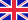•# Theory of Duality in Mathematical Programming

Autor: Manfred Walk

Courses on mathematical programming are now part of standard teaching programs of universities and institutes. The aim of this book is to introduce students of mathematics, economics, technology and other related subjects to the qualitative theory of mathematical... Viac o knihe

Na objednávku, dodanie 2-4 týždne

51.42 €

bežná cena: 60.49 €

## O knihe

Courses on mathematical programming are now part of standard teaching programs of universities and institutes. The aim of this book is to introduce students of mathematics, economics, technology and other related subjects to the qualitative theory of mathematical programming in paired vector spaces. Prerequisite for the study of this book is only a basic knowledge of analysis, of elements of functional analysis and linear algebra. The application of elementary ideas of functional analysis is convenient for a more rigorous construction of proofs and for some generalizations of the finite dimensional theory on infinite dimensional Banach-spaces. An important feature of this book is the use of a principle of duality to formulate the theoretical basis of many different concrete programming problems. The main idea of the book is to present relations of duality and to construct a general theoretical basis for different special programming problems.

• Vydavateľstvo: Springer Vienna
• Rok vydania: 1989
• Formát: Hardback
• Rozmer: 260 x 183 mm
• Jazyk: Anglický jazyk
• ISBN: 9783211820575

Generuje redakčný systém BUXUS CMS spoločnosti ui42.